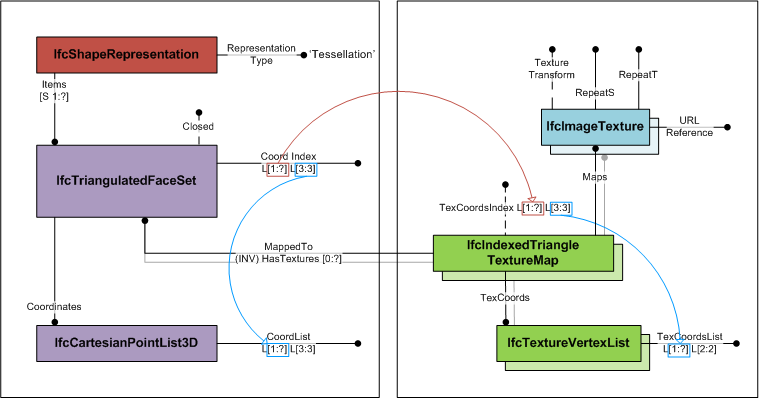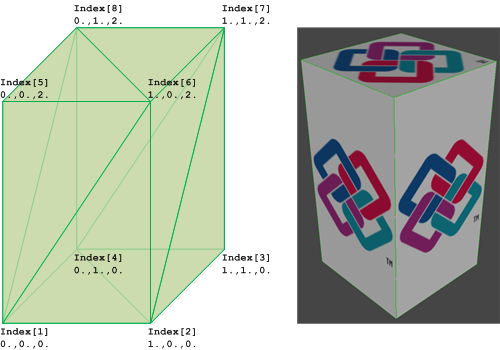# IFC 4.3.x development

## 8.12.3.20 IfcIndexedTriangleTextureMap

### 8.12.3.20.1 Semantic definitions at the entity

The IfcIndexedTriangleTextureMap provides the mapping of the 2-dimensional texture coordinates to the surface onto which it is mapped. It is used for mapping the texture to triangles of the IfcTriangulatedFaceSet.

The IfcIndexedTriangleTextureMap defines an index into an indexed list of texture coordinates. The TexCoordIndex is a two-dimensional list, where

• first dimension is the unbounded list of faces corresponding to the list of triangles defined by CoordIndex at IfcTriangulatedFaceSet;
• second dimension is the fixed list of three indices to texture vertices cooresponding to the fixed list of indices to vertices at IfcTriangulatedFaceSet

The TexCoords defined at supertype IfcIndexedTextureMap are a two-dimensional list of texture coordinates provided by two parameter values. Each index of the second dimension list of TexCoordIndex points to a texture vertex in TexCoords.

Figure 1 shows the use of IfcTriangulatedFaceSet with textures.Figure 1 — Indexed triangle texture map

Figure 2 illustrates an IfcTriangulatedFaceSet represented by
IfcTriangulatedFaceSet.CoordIndex: ((1,6,5), (1,2,6), (6,2,7), (7,2,3), (7,8,6), (6,8,5), (5,8,1), (1,8,4), (4,2,1), (2,4,3), (4,8,7), (7,3,4))
IfcCartesianPointList.CoordList: ((0.,0.,0.), (1.,0.,0.), (1.,1.,0.), (0.,1.,0.), (0.,0.,2.), (1.,0.,2.), (1.,1.,2.), (0.,1.,2.))
IfcIndexedTriangleTextureMap.TexCoordsIndex: ((1 4 3), (1 2 4), (3 1 4), (4 1 2), (8 7 6), (6 7 5), (4 3 2), (2 3 1), (5 8 7), (8 5 6), (2 4 3), (3 1 2))
IfcTextureVertexList.TexCoordsList: ((0. -0.5), (1. -0.5), (0. 1.5), (1. 1.5), (0. 0.), (0. 1.), (1. 0.), (1. 1.))Figure 2 — Indexed triangle texture map geometry

HISTORY  New entity in IFC4.

### 8.12.3.20.1 Attributes

#Attribute Type Description
IfcPresentationItem
IfcTextureCoordinate
1Maps LIST [1:?] OF IfcSurfaceTexture Reference to the one (or many in case of multi textures with identity transformation to geometric surfaces) subtype(s) of IfcSurfaceTexture that are mapped to a geometric surface by the texture coordinate transformation.
IfcIndexedTextureMap
2MappedTo IfcTessellatedFaceSet Reference to the IfcTessellatedFaceSet to which it applies the texture map.
3TexCoords IfcTextureVertexList Indexable list of texture vertices.
IfcIndexedTriangleTextureMap
4TexCoordIndexOPTIONAL LIST [1:?] OF LIST [3:3] OF IfcPositiveIntegerIndex into the IfcTextureVertexList for each vertex of the triangles representing the IfcTriangulatedFaceSet.

### 8.12.3.20.3 Formal representations

```ENTITY IfcIndexedTriangleTextureMap
SUBTYPE OF (IfcIndexedTextureMap);
TexCoordIndex : OPTIONAL LIST [1:?] OF LIST [3:3] OF IfcPositiveInteger;
END_ENTITY;```